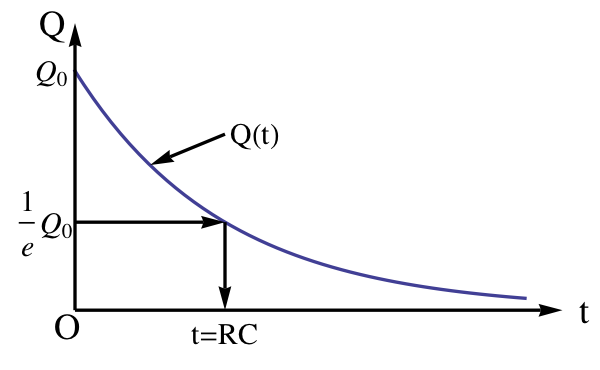## Section37.1Capacitor Discharging Circuit

A charged capacitor provides a ready supply of separated charges. When you provide a conducting path for excess electrons on the negative plate to drift to positive plate, it leads to discharge of the capacitor. This process releases electrical energy in a short time.

To use charges on a charged capacitor one can connect the ends of the capacitor through a device of resistance $R$ and a switch S as indicated in Figure 37.1.1. When the switch is closed, current flows in the circuit until electrons from the negative plate neutralize the positive charges on the positive plate.

Rate of discharge depends on the current value of charges on the plates, which leads to charge decaying exponentially. Let $Q_0$ be the starting charge at $t=0\text{.}$ Then, using Calculus, it will be shown below that charge remaining on capacitor at time $t$ will be

\begin{equation*} Q(t) = Q_0 e^{ - t/RC}. \end{equation*}

A plot of this function versus time is shown in Figure 37.1.3. The rate of discharge, i.e., current in the circuit varies with time and is proportional to the charge on the plates at the instant.

\begin{equation*} \text{Rate of discharge } = \dfrac{1}{RC}\, Q(t). \end{equation*}

The time to reach $\dfrac{1}{e}$ of the original charge, where $e$ is the Euler number with value $e = 2.718...\text{,}$ is an important characteristic of the circuit. This time is called time constant of the circuit. The time constant is usually denoted by the Greek letter $\tau\text{.}$ Of course, during this time, $1-1/e$ of the charge, which is approximately two-thirds of the charge, has been discharged through the circuit. The time constant of the circuit in Figure 37.1.1 is product of the resistance and capacitance of the circuit.

\begin{equation} \tau = RC.\label{eq-cap-discharging-circuit-tau}\tag{37.1.1} \end{equation}

By adjusting $R$ we can congtrol the rate at which dischsrging of a capacitor can take place. In another section, we will study charging process. We will find there that the rate of charging has also the same time constant.

### Subsection37.1.1(Calculus) Equation of Motion for Discharging a Capacitor

Let us examine discharging circuit in Figure 37.1.1 at instant $t\text{.}$ Let $I(t)$ be current in the circuit, $\pm Q(t)$ be charges on the plates. The potential drop $V_c$ across the capacitor is given by the capacitor equation.

\begin{equation} V_c(t) = \dfrac{Q(t)}{C}.\label{eq-cap-discharging-circuit-Q-eq-CV}\tag{37.1.2} \end{equation}

By Kirchhoff's Loop Rule on the loop a-b-a' in Figure 37.1.2, we find the following equation for relation among these quantities.

\begin{equation} -R I + V_c = 0.\label{eq-cap-discharging-circuit-KVL-loop-eq}\tag{37.1.3} \end{equation}

Current $I$ is rate at which charge is decreasing in time. Therefore,

\begin{equation} I = - \dfrac{dQ}{dt}.\label{eq-cap-discharging-circuit-I-eq-dQdt}\tag{37.1.4} \end{equation}

From Eqs. (37.1.2) to (37.1.4) gives the following equations for rate at which charge drops in time.

\begin{equation} \dfrac{dQ}{dt} = - \dfrac{1}{RC}\, Q.\label{eq-cap-discharging-circuit-eom}\tag{37.1.5} \end{equation}

Let $\pm Q_0$ be the charges on capcitor plates at $t=0\text{,}$ when the circuit switch was closed. Then, Eq. (37.1.5) can be solved to show that charge at an arbitrary instant $t \gt 0$ will be

\begin{equation} Q(t) = Q_0\, e^{- t / RC}.\label{eq-cap-discharging-circuit-eom-soln-Q}\tag{37.1.6} \end{equation}

A plot of $Q$ versus $t$ presented in Figure 37.1.3 shows the exponential decaying behavior of the discharge process.Figure 37.1.3. Charge on positive plate decays exponentially with time with a time constant $\tau= RC\text{.}$ The time constant is equal to the time for charge to decay to $1/e$ of its initial value.

Plots of current $I$ vs $t$ and potential drop across the capacitor $V_c$ vs $t$ show similar plots.

\begin{align*} I \amp = -\dfrac{dQ}{dt} = \dfrac{Q_0}{RC}\, e^{- t / RC}.\\ V_c \amp = \dfrac{Q}{C} = \dfrac{Q_0}{C}\, e^{- t / RC}. \end{align*}

Consider a capacitor of capacitance $2\;\mu\text{F}\text{.}$ (a) Find the time constant of a circuit when the capacitor is connected to a $1.0\text{ k}\Omega$ resistor. (b) Suppose the capacitor was charged to contain $5.0\;\mu\text{C}$ at $t = 0\text{.}$ At what time will there be $2.0\;\mu\text{C}$ left on the capacitor?

Hint

Use the solution of discharging circuit.

(a) $2\text{ ms} \text{,}$ (b) $1.8\text{ ms} \text{.}$

Solution 1 (a)

(a) The time constant follows from the result $\tau = RC$ for this circuit given above.

\begin{equation*} \tau = RC = 1000\ \Omega\times 2\times 10^{-6}\text{ F} = 2\times 10^{-3}\text{ sec} = 2\text{ ms}. \end{equation*}
Solution 2 (b)

(b) Using (37.1.6), we set up the following equation for charge at an unknown time.

\begin{equation*} 2\ \mu\text{C} = 5\ \mu\text{C} \times \exp{\left(-\frac{t}{2\text{ ms} } \right)} \end{equation*}

Therefore, we have the following exponential equation to solve.

\begin{equation*} \exp{\left(-\frac{t}{2 \text{ms} } \right)} = 0.4. \end{equation*}

Taking natural logarithm of both sides converts this equation into a linear equation for the unknown $t\text{.}$

\begin{equation*} -\frac{t}{2 \text{ ms} } = \ln(0.4), \end{equation*}

which gives $t=1.8\text{ ms}\text{.}$